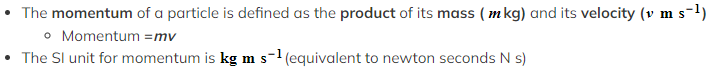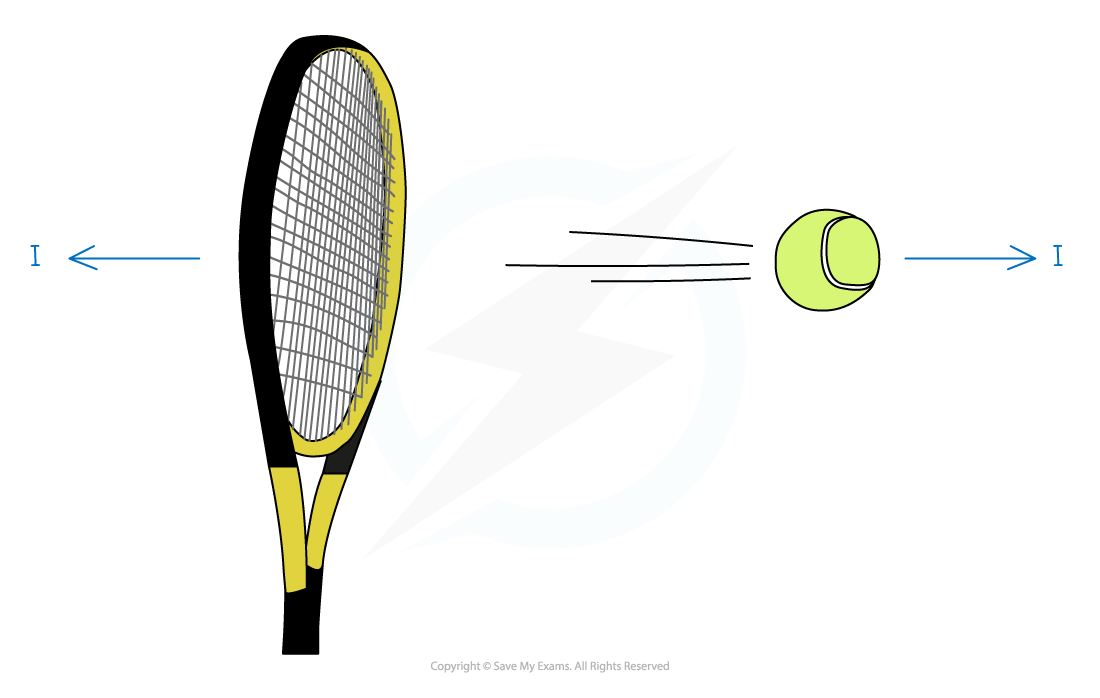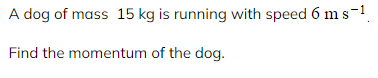# CIE A Level Maths: Mechanics复习笔记5.1.1 Momentum

### Momentum

#### What is momentum?

• Any object that has mass and is moving has momentum
• Momentum measures the quantity of motion that an object has
•Momentum is a vector quantity - so it has a magnitude and direction
• The direction of the momentum of a particle is the same as the direction of motion of the particle
• The momentum is negative if the velocity is negative
• If an object is falling vertically then momentum will be downwards

#### What happens when two objects are in contact?

• If two objects are in contact with each other then by Newton’s Third Law there will be equal and opposite reaction forces
• One object gains momentum from the impact and the other loses momentum
• The change in momentum will be equal for each particle provided there are no external forces
• Remember momentum is a vector and can be negative
• For example, consider the scenario where a tennis ball is struck by a racket, suppose the momentum of the racket and the ball before the impact is 5 kg m s-1  and  -3 kg m s-1  and the momentum of the racket afterwards is -2 kg m s-1
• This means the racket has a loss of 7 kg m s-1 in momentum
• Therefore the ball has a gain of 7 kg m s-1 in momentum
• Therefore the momentum of ball after the impact is 4 kg m s-1#### Worked Example